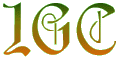http://IanGClark.net/

# Ian's Puzzles and Problems to Think About page

My Puzzles
Good Puzzles I have seen
• Write all the integers from 0 to 100 using four 4's and mathematical symbols only. For instance, 0 = 4 + 4 - 4 - 4; 1 = 44/44; and so on. (Symbols: +, -, *, /, (, ), !, sqrt, ^, .). (There is often more than one answer for each number!. I had lots of fun with this - then I put 'four fours problem' in to Google and founds many sites listing the answers - including some which list 0 to 1000!).

• A new building has 8 floors and several lifts. Each lift serves only 3 floors. What is the minimum number of lifts required so that from any floor you can reach any other floor using only 1 lift? (There are several solutions to this problem - I think I have found the smallest)

• BrainBashers - Brain Teazers and Puzzles.
• Puzzle World.
Hmmmmm....
• Why is it that doctors call what they do practice?
• If con is the opposite of pro, is Congress the opposite of progress?
• What if there were no hyperthetical questions?